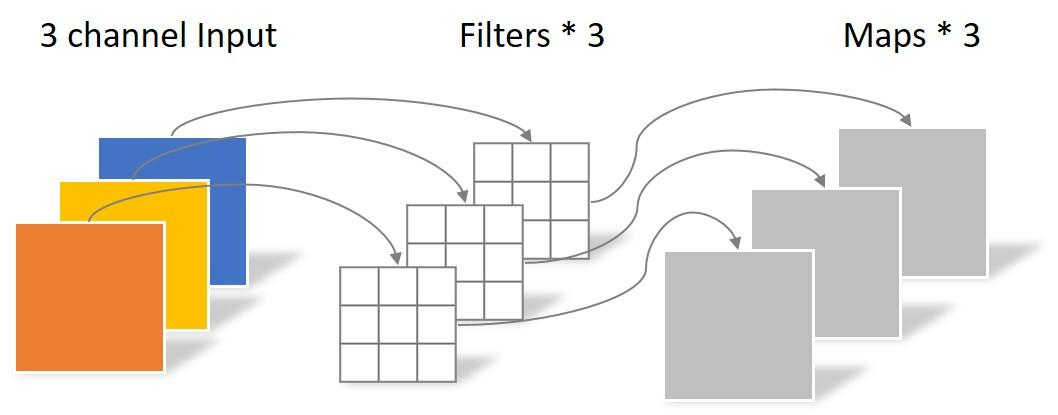## 常规卷积操作

N_std = 4 × 3 × 3 × 3 = 108

## Depthwise Separable Convolution

Depthwise Separable Convolution是将一个完整的卷积运算分解为两步进行，即Depthwise Convolution与Pointwise Convolution。

### Depthwise Convolution

N_depthwise = 3 × 3 × 3 = 27

Depthwise Convolution完成后的Feature map数量与输入层的通道数相同，无法扩展Feature map。而且这种运算对输入层的每个通道独立进行卷积运算，没有有效的利用不同通道在相同空间位置上的feature信息。因此需要Pointwise Convolution来将这些Feature map进行组合生成新的Feature map。

### Pointwise Convolution

Pointwise Convolution的运算与常规卷积运算非常相似，它的卷积核的尺寸为 1×1×M，M为上一层的通道数。所以这里的卷积运算会将上一步的map在深度方向上进行加权组合，生成新的Feature map。有几个卷积核就有几个输出Feature map。如下图所示。

N_pointwise = 1 × 1 × 3 × 4 = 12

## 参数对比

N_std = 4 × 3 × 3 × 3 = 108

Separable Convolution的参数由两部分相加得到：
N_depthwise = 3 × 3 × 3 = 27
N_pointwise = 1 × 1 × 3 × 4 = 12
N_separable = N_depthwise + N_pointwise = 39

https://blog.csdn.net/tintinetmilou/article/details/81607721blog.csdn.net